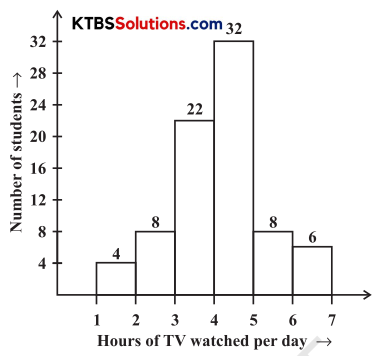# KSEEB Solutions for Class 8 Maths Chapter 5 Data Handling Ex 5.1

## KSEEB Solutions for Class 8 Maths Chapter 5 Data Handling Ex 5.1

Question 1.
For which of these would you use a histogram to show the data?
(a) The number of letters for different areas in a postman’s bag.
(b) The height of competitors in an athletics meet.
(c) The number of cassettes produced by 5 companies.
(d) The number of passengers boarding trains from 7:00 a.m. to 7:00 p.m. at a station.
Give reasons for each.
Solution:
(a) Histogram, to show that data is possible only when class-interval of the data can be formed. In case, when the letters to different areas are to be delivered, the class interval is not possible. Hence, these cannot be represented by a histogram. But these data can be represented by bar-graph.

(b) Yes, it can be represented by a histogram. Because through the data of height we can form class-interval.(c) No, these data can’t be represented by a histogram. As the data can’t be represented by class intervals.

(d) Yes, these data can be represented by a histogram, as the class interval can be formed.

Question 2.
The shoppers who come to a departmental store are marked as man (M), woman (W), boy (B) or girl (G). The following list gives the shoppers who came during the first hour in the morning:
W W W G B W W M G G M M W W W W G B M W B G G M W W M M W W W M W B W G M W W W W G W M M W W M W W IV M G W M M B G G W
Make a frequency distribution table using tally marks. Draw a bar graph to illustrate it.
Solution:
Frequency Distribution Table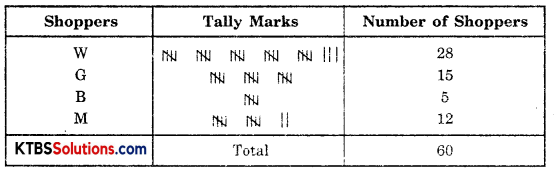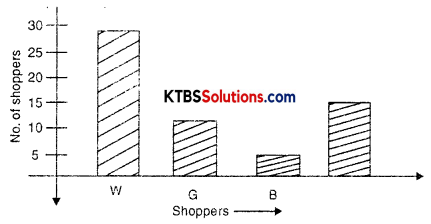Question 3.
The weekly wages (in Rs) of 30 workers in a factory are.
830, 835, 890, 810, 835, 836, 869, 845, 898, 890, 820, 860, 832, 833, 855, 845, 804, 808, 812, 840, 885, 835, 835, 836, 878, 840, 868, 890, 806, 840
Using tally marks, make a frequency table with intervals as 800 – 810, 810 – 820 and so on.
Solution:
Frequency Distribution Table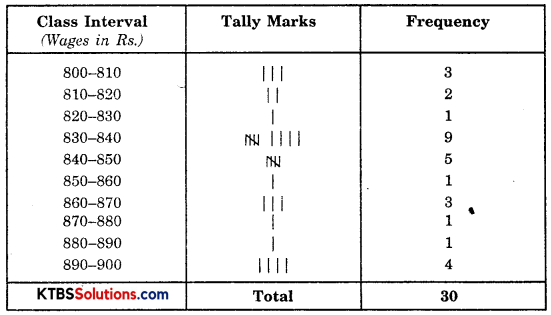Question 4.
Draw a histogram for the frequency table made for the data in Question 3, and answer the following questions: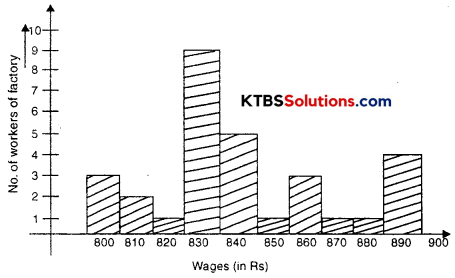(i) Which group has the maximum number of workers?
(ii) How many workers earn Rs 850 and more?
(iii) How many workers earn less than Rs 850?
Solution:
(i) Group 830-840 has a maximum number of workers.
(ii) Number of workers whose earning is Rs 850 and more = 1 + 3 + 1 + 1 + 4 = 10
(iii) Number of workers whose earning less than 850 = 3+ 2 + 1 + 9 + 5 = 20Question 5.
The number of hours for which students of a particular class watched television during holidays is shown through the given graph.